# Gauss Elimination Method

Gauss elimination method is used to solve a system of linear equations. Let’s recall the definition of these systems of equations. A system of linear equations is a group of linear equations with various unknown factors. As we know, unknown factors exist in multiple equations. Solving a system involves finding the value for the unknown factors to verify all the equations that make up the system.

If there is a single solution that means one value for each unknown factor, then we can say that the given system is a consistent independent system. If multiple solutions exist, the system has infinitely many solutions; then we say that it is a consistent dependent system. If there is no solution for unknown factors, and this will happen if there are two or more equations that can’t be verified simultaneously, then we say that it’s an inconsistent system.

This can be summarized in a table as given below:

 Name of the system of equations Number of solutions Consistent independent system 1 Consistent dependent system Multiple or Infinitely many Inconsistent system 0

Now, let’s have a look at the method that can be used to find the solution(s) of the given system of equations.

## What is the Gauss Elimination Method?

In mathematics, the Gaussian elimination method is known as the row reduction algorithm for solving linear equations systems. It consists of a sequence of operations performed on the corresponding matrix of coefficients. We can also use this method to estimate either of the following:

• The rank of the given matrix
• The determinant of a square matrix
• The inverse of an invertible matrix

To perform row reduction on a matrix, we have to complete a sequence of elementary row operations to transform the matrix till we get 0s (i.e., zeros) on the lower left-hand corner of the matrix as much as possible. That means the obtained matrix should be an upper triangular matrix. There are three types of elementary row operations; they are:

• Swapping two rows and this can be expressed using the notation ↔, for example, R2 ↔ R3
• Multiplying a row by a nonzero number, for example, R1 → kR2 where k is some nonzero number
• Adding a multiple of one row to another row, for example, R2 → R2 + 3R1

The obtained matrix will be in row echelon form. The matrix is said to be in reduced row-echelon form when all of the leading coefficients equal 1, and every column containing a leading coefficient has zeros elsewhere. This final form is unique; that means it is independent of the sequence of row operations used. We can understand this in a better way with the help of the example given below.

## Gauss Elimination Method with Example

Let’s have a look at the gauss elimination method example with a solution.

Question:

Solve the following system of equations:

x + y + z = 2

x + 2y + 3z = 5

2x + 3y + 4z = 11

Solution:

Given system of equations are:

x + y + z = 2

x + 2y + 3z = 5

2x + 3y + 4z = 11

Let us write these equations in matrix form.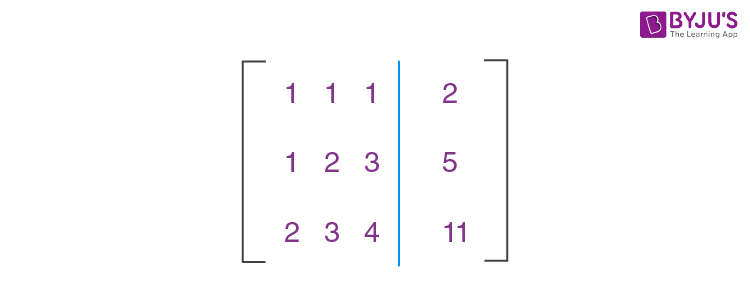Subtracting R1 from R2 to get the new elements of R2, i.e. R2 → R2 – R1.

From this we get,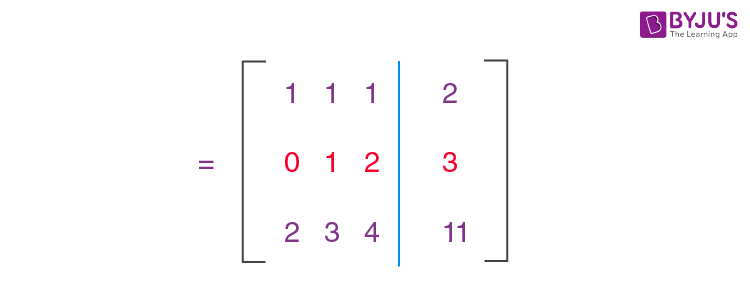Let us make another operation as R3 → R3 – 2R1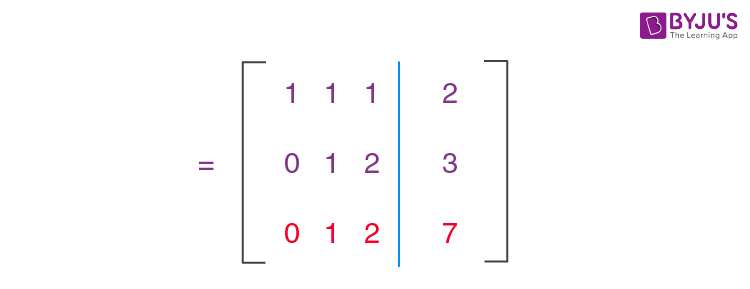Subtract R2 from R1 to get the new elements of R1, i.e. R1 → R1 – R2.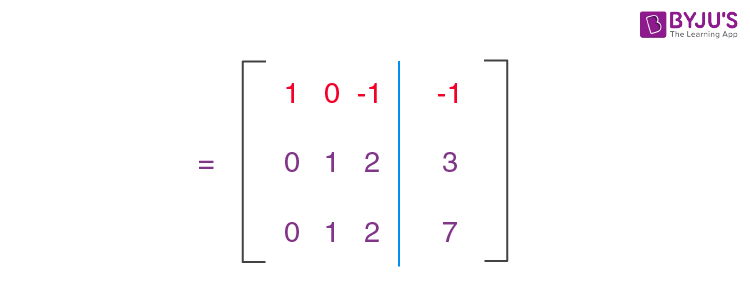Now, subtract R2 from R3 to get the new elements of R3, i.e. R3 → R3 – R2.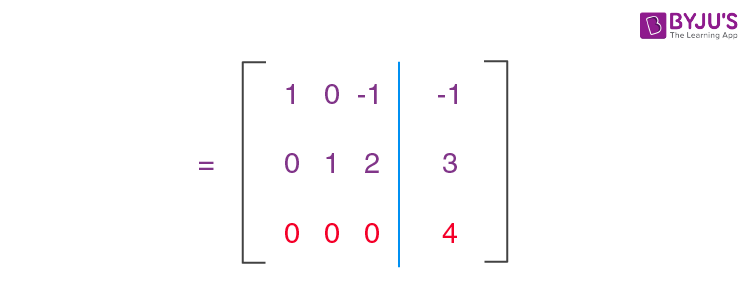Here,

x – z = -1

y + 2z = 3

0 = 4

That means, there is no solution for the given system of equations.

### Related Articles:

Cramer’s Rule LU Decompositions
Jacobian Method Fixed Point Iteration Method
Newton Raphson Method Runge-Kutta RK4 Method

### Gauss Elimination Method Problems

1. Solve the following system of equations using Gauss elimination method.

x + y + z = 9

2x + 5y + 7z = 52

2x + y – z = 0

2. Solve the following linear system using the Gaussian elimination method.

4x – 5y = -6

2x – 2y = 1

3. Using Gauss elimination method, solve:

2x – y + 3z = 9

x + y + z = 6

x – y + z = 2

## Frequently Asked Questions on Gauss Elimination Method

Q1

### Why Gauss elimination method is used?

The Gauss elimination method is used for solving a given system of linear equations. Other methods of solving system of linear equations are the Jacobi method, Cramer’s rule, Gauss-Seidel method etc.

Q2

### What type of method is the Gauss elimination method?

The Gauss Elimination method is a direct method for solving a system of linear equations because, within a finite number of determined steps, we can solve the given system of linear equations.

Q3

### What are the steps of the Gauss elimination method?

The steps of the Gauss elimination method are
(1) Write the given system of linear equations in matrix form AX = B, where A is the coefficient matrix, X is a column matrix of unknowns and B is the column matrix of the constants.
(2) Reduce the augmented matrix [A : B] by elementary row operations to get [A’ : B’].
(3) We get A’ as an upper triangular matrix.
(4) By the backward substitution in A’X = B’, we get the solution of the given system of linear equations.

Q4

### How Gauss-Jordan Method is different from the Gauss-Elimination method?

Both Gauss-Jordan and Gauss elimination are somewhat similar methods, the only difference is in the Gauss elimination method the matrix is reduced into an upper-triangular matrix whereas in the Gauss-Jordan method is reduced into a diagonal matrix.